https://doi.org/10.5194/acp-18-15903-2018
https://doi.org/10.5194/acp-18-15903-2018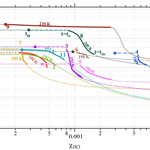# The influence of HCl on the evaporation rates of H2O over water ice in the range 188 to 210 K at small average concentrations

Christophe Delval and Michel J. Rossi
Abstract

The evaporation flux Jev(H2O) of H2O from HCl-doped typically 1.5 µm or so thick vapor-deposited ice films has been measured in a combined quartz crystal microbalance (QCMB)–residual gas mass spectrometry (MS) experiment. Jev(H2O) has been found to show complex behavior and to be a function of the average mole fraction χHCl of HCl in the ice film ranging from 6×1014 to 3×1017 molecule cm−2 s−1 at 174–210 K for initial values ${\mathit{\chi }}_{\mathrm{HCl}}^{\mathrm{0}}$ ranging from $\mathrm{5}×{\mathrm{10}}^{-\mathrm{5}}$ to $\mathrm{3}×{\mathrm{10}}^{-\mathrm{3}}$ at the start of the evaporation. The dose of HCl on ice was in the range of 1 to 40 formal monolayers and the H2O vapor pressure was independent of χHCl within the measured range and equal to that of pure ice down to 80 nm thickness. The dependence of Jev(H2O) with increasing average χHCl was correlated with (a) the evaporation range rb∕e parameter, that is, the ratio of Jev(H2O) just before HCl doping of the pure ice film and Jev(H2O) after observable HCl desorption towards the end of film evaporation, and (b) the remaining thickness dD below which Jev(H2O) decreases to less than 85 % of pure ice. The dependence of Jev(H2O) with increasing average χHCl from HCl-doped ice films suggests two limiting data sets, one associated with the occurrence of a two-phase pure ice/crystalline HCl hydrate binary phase (set A) and the other with a single-phase amorphous HCl∕H2O binary mixture (set B). The measured values of Jev(H2O) may lead to significant evaporative lifetime extensions of HCl-contaminated ice cloud particles under atmospheric conditions, regardless of whether the structure corresponds to an amorphous or crystalline state of the HCl∕H2O aggregate.

Share
Dates
1 Introduction

HCl is among the mineral acids that control the acidity of the atmosphere, together with HNO3 and H2SO4. The production of atmospheric HCl predominantly takes place in the middle and upper stratosphere where O3 is formed owing to photolysis of halogen-containing source gases such as CFCs (chlorofluorocarbons). However, there are no known sources of HCl in the upper troposphere (UT) because scavenging processes of HCl throughout the troposphere are very efficient, which leads to HCl background concentrations of less than 0.1 ppb (Graedel and Keene, 1995). The absence of significant sources in the troposphere, the long photolytic lifetime of HCl and the fact that the production region is well separated from the regions of interest, namely the UT and the lower stratosphere (LS), all contribute to the fact that HCl is an excellent tracer for stratospheric ozone in the UT (Marcy et al., 2004). Owing to the frequent occurrence of cirrus clouds in this atmospheric region it is of obvious interest to study the interaction of HCl with atmospheric ice particles at relevant temperature and pressure conditions (Jensen et al., 2001; Zerefos et al., 2003). The compact correlation between O3 and HCl has been used to monitor stratospheric–tropospheric exchange processes and stratospheric O3 intrusions into the troposphere that are still an active field of investigation (Houghton et al., 2001).

HCl is of importance in the LS as it partakes in heterogeneous reactions on polar stratospheric ice clouds (PSCs) as well as on background stratospheric H2SO4 aerosol according to the following reaction taken as an example:

$\begin{array}{}\text{(R1)}& \mathrm{HCl}\left(\mathrm{ads}\right)+{\mathrm{ClONO}}_{\mathrm{2}}{\mathrm{Cl}}_{\mathrm{2}}\left(\mathrm{g}\right)+{\mathrm{HNO}}_{\mathrm{3}}\left(\mathrm{ads}\right).\end{array}$

These reactions efficiently convert inactive Cl-containing reservoir molecules such as HCl and ClONO2 into active photolyzable Cl-containing compounds in a single reaction. Typical examples of such photolabile reaction products are Cl2, ClNO2 and HOCl that will change the atmospheric composition owing to the high reactivity of the photolysis products such as atomic Cl (Solomon et al., 1986; Tolbert et al., 1987; WMO, 2003). It thus follows that HCl is of stratospheric importance and is frequently used as a model compound for heterogeneous reactions on ices that has inspired many laboratory kinetic studies (Leu et al., 1991; Hanson and Ravishankara, 1992; Chu et al., 1993; Flückiger et al., 1998; Hynes et al., 2001; Abbatt, 2003).

HCl forms hydrates of variable stoichiometry when exposed to ice depending on the temperature of deposition and the partial pressure of HCl (Graham and Roberts, 1997; Ortega et al., 2004). X-ray diffraction has allowed the identification of four crystalline hydrates containing one (Yoon and Carpenter, 1959), two (Lundgren and Olovson, 1967), three (Lundgren and Olovson, 1967a) and six (Taesler and Lundgren, 1978) H2O per HCl molecule. In addition, amorphous mono-, tetra- and hexa-hydrates have been reported under various experimental conditions (Yoon and Carpenter, 1959; Delzeit et al., 1993a). The control of growth conditions of a specific HCl hydrate is sometimes elusive, but the formation of a saturated HCl hexahydrate phase has been reported at sufficiently large HCl exposure (Graham and Roberts, 1995) using amorphous ice as a starting point despite the fact that the hexahydrate is said to nucleate with difficulty, at least in thin films (Ortega et al., 2004). However, the molecular and dynamic details of the crystallization process have not been investigated as yet.

Fourier transform IR (FTIR) absorption measurements have enabled the characterization of both amorphous as well as crystalline HCl hydrates at growth conditions that are sometimes significantly different compared to the samples investigated using X-ray diffraction. Vibrational spectra of HCl hydrates in the mid-IR have been routinely used for identification purposes for some time (Ferriso and Hornig, 1955; Gilbert and Sheppard, 1973). Recently, the mid-IR absorption spectra of the four HCl hydrates mentioned above have been assigned in a comprehensive and definitive way, albeit without simultaneous proof of the crystalline structure using X-ray diffraction (Buch et al., 2002; Xueref and Dominé, 2003). More recently, the reflection absorption IR spectrum (RAIR) of crystalline HCl hexahydrate in the mid-IR range has been recorded and assigned using theoretical calculations based on density functional theory that results in a refinement of the geometric structure of the HCl hydrates and a prediction of the vibrational modes of the crystal (Ortega et al., 2004). It must be recalled that FTIR spectra in transmission and reflection may in most cases not be directly compared across the mid-IR range.

Although theoretical electronic structure calculations predict spontaneous ionization of adsorbed HCl (Gertner and Hynes, 1996; Bolton and Petterson, 2001), most experiments point towards a seemingly thermally activated ionization process that may be enabled by structural factors of the ice matrix that are themselves a function of temperature. Consistent with these results concentration profiling experiments of HCl/ice adsorbates using static secondary ionization mass spectroscopy (SIMS) techniques failed to discover molecularly adsorbed HCl on ice in the range 90–150 K (Donsig and Vickerman, 1997). In conclusion, both experimental and theoretical studies clearly point to the absence of significant quantities of molecularly or covalently adsorbed HCl under stratospheric conditions. Instead, HCl is ionized and solvated by H2O on the surface of ice films and may occur either as amorphous HCl∕H2O hydrates of undefined stoichiometry or as crystalline HCl hydrates. However, these facts do not rule out the presence of small amounts of molecularly adsorbed HCl on ice that may be intermediates in the complex mechanism of HCl adsorption on ice, as evidenced by the negative temperature dependence of the rate of uptake of HCl on ice (Flückiger et al., 1998). In fact, such an intermediate has been invoked in the description of HCl adsorption on ice under atmospheric conditions using a chemical kinetic model based on a multitude of experimental observables collected upon HCl uptake on ice (Flückiger and Rossi, 2003).

Work by Parent and coworkers uses near-edge X-ray absorption spectroscopy (NEXAFS) of HCl-doped low-temperature ice substrates in order to determine the relative population of ionic and covalently bound HCl and distinguish between bulk and HCl surface states in the temperature range 20 to 150 K (Bournel et al., 2002; Parent and Laffon, 2005). The results seem to confirm the consensus on the low-temperature existence of molecularly adsorbed HCl up to 90 K beyond which an increasing amount of HCl is converted into an ionic form, such as ${\mathrm{H}}_{\mathrm{3}}{\mathrm{O}}^{+}{\mathrm{Cl}}^{-}$ (Eigen cation) or ${\mathrm{H}}_{\mathrm{5}}{\mathrm{O}}_{\mathrm{2}}^{+}{\mathrm{Cl}}^{-}$ (Zundel cation) formed by spontaneous ionization of adsorbed HCl on ice, up to completion at 150 K (Buch et al., 2008). The newest work by Parent compares NEXAFS with photoemission (UPS, XPS) and FTIR in transmission of thin HCl∕H2O films (Parent et al., 2011). The results are roughly consistent but surprising in the sense that these workers find 92 % ionically dissolved HCl in/on ice at 50 K in contrast to Kang et al. (2000) and Devlin et al. (2000) under similar exposure (dose) and temperature conditions. In addition, Parent et al. (2011) perform the NEXAFS experiment on a (thick) 100 ML “crystalline” H2O ice substrate deposited at 150 K, whereas the photoemission and FTIR absorption experiments used a 4 ML thin ice slab deposited at 120 K. The question has to be raised whether the two types of used ice films may be responsible for some of the discrepancies in the results because both the density and the structure of ice are known to be a strong function of temperature and deposition conditions (Kuhs et al., 2012; Schriver-Mazzuoli et al., 2000). The most recent work of Parent et al. (2011) sparked an interesting controversy in the assignment of the FTIR absorption spectrum of thin HCl∕H2O films and led to two comments showcasing the difficulties of intercomparison of nominally identical experiments (Devlin and Kang, 2012; Parent et al., 2012).

Furthermore, the results indicate that the “dangling bonds” of the ice surface attributed to isolated OH groups are not the unique site of HCl adsorption, even in the range 20–90 K (Flückiger and Delval, 2002). The present work suggests that maiden uptake of HCl onto pure ice weakens and perturbs the crystal structure of the ice matrix in an irreversible way such that additional sites for HCl adsorption and ionization are created akin to Parent et al. (2011). Initial HCl uptake on pure ice therefore has a catalytic effect on the following HCl uptake. This irreversible nature of initial HCl dosing has been known for several years and was observed some time ago in Knudsen flow reactor studies on the HCl∕H2O system under steady-state conditions of both HCl and H2O at temperatures representative of the UT/LS (Flückiger et al., 1998; Oppliger et al., 1997). The most recent experimental work on HCl∕H2O at an atmospherically relevant (“warm”) temperature (253 K) has examined the HCl depth profile using XPS spectroscopy and finds molecularly adsorbed (physisorbed) HCl at its outermost layer and ionic dissociation in deeper layers (Kong et al., 2017). Complementary X-ray absorption results also point towards a perturbation of the crystal structure of ice in the aftermath of HCl adsorption/dissolution into deeper layers of ice.

Table 1Hardware parameters of both cryogenic sample supports of HCl-doped ice.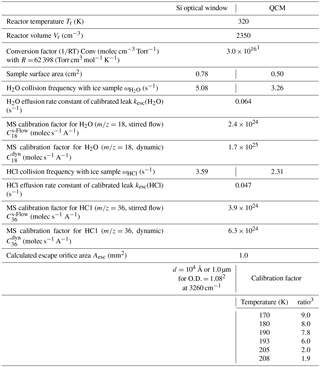1 Wall temperature of the reactor at T=320 K. 2 See Delval et al. (2003). 3 Corresponds to the ratio between the true number of molecules present on the QCM support and the number of molecules displayed by the IC5 controller (Delval et al., 2004).

We have concluded from recent work that HCl doping in quantities of a submonolayer to several monolayers of HCl leads to the decrease in both the evaporative flux Jev (molecule cm−2 s−1) or rate Rev (molecule cm−3 s−1) and the rate of condensation kcond (s−1), of H2O in the presence in ice without perturbing the equilibrium vapor pressure of H2O, ${P}_{{\mathrm{H}}_{\mathrm{2}}\mathrm{O}}^{\text{eq}}$ (Delval et al., 2003). We have furthermore shown that the way Jev of H2O decreases with time depends on the rate of deposition or the integral of deposited HCl, namely RHCl (molecule s−1) and NHCl (molecule), respectively. It appears that two observed HCl species on/in ice, namely single-phase amorphous HCl∕H2O mixtures and a binary phase consisting of pure ice and an as yet unidentified crystalline HCl hydrate, HCl⋅xH2O, decrease Jev(H2O) to a different extent, as proposed in Delval et al. (2003). These results have led us to perform systematic experiments in this work using the quartz crystal microbalance (QCMB) combined with residual gas mass spectrometry (MS) that we have used successfully in the past (Delval and Rossi, 2004) in order to investigate the temporal change in Jev(H2O) with the increasing average mole fraction of HCl, χHCl, remaining in the ice. One of the goals of the present work is to determine the influence of the HCl deposition parameters on the temporal change in Jev and the mass accommodation coefficient α during evaporation of a HCl-doped ice film and its consequence for the lifetime of atmospheric ice particles contaminated by HCl. This issue is key in relation to the importance of heterogeneous vs. homogeneous atmospheric reactions at midlatitudes, as has been pointed out in the past (Solomon et al., 1986, 1997).

2 Experimental

The emphasis of the present experiments was placed on the deposition of small amounts of HCl ranging in doses from 1 to 40 formal monolayers of HCl where a formal monolayer of adsorbed HCl corresponded to a surface concentration of 2.5×1014 molecule cm−2 (Table A1), which is a consensus value obtained from several selected experiments. The apparatus as well as the methods used for calibration and the HCl deposition procedure have been described in detail elsewhere (Delval and Rossi, 2005). The experimental conditions are generally identical to the ones presented in Delval and Rossi (2005) and the instrumental parameters are summarized in Table 1. The only significant difference between the study of HNO3-doped ice and the present condensed-phase investigation of HCl-doped ice lies in the mode of trace gas admission. HCl was deposited by backfilling the reactor under stirred flow conditions with the inlet tubing used for trace gas injection oriented towards one side of the Si window of the cryostat set at ambient temperature, whereas HNO3 was deposited by directed injection onto ice films supported by the quartz crystal of the QCMB as referenced above. Evaporation experiments have been performed isothermally on samples in the temperature range 174–210 K under dynamic pumping conditions, that is, at maximum pumping speed (gate valve open) in order to prevent readsorption of HCl on the ice substrate.

Table 2Representative experimental results for the kinetics of H2O evaporation in the presence of HCl for increasing HCl deposition temperatures at given rates of deposition RHCl and doses of HCl ${N}_{\mathrm{HCl}}^{\mathrm{dep}}$. In the first column the number refers to the corresponding experiment and identifies the data displayed in Fig. 2.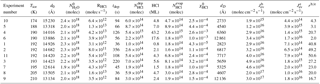First, an approximately 1.5 µm thick ice film was grown at 190 K on the quartz crystal of the QCMB by deposition of bidistilled water vapor at a rate of 1×1017 molecule cm−2 s−1 under static conditions. The H2O equilibrium vapor pressure agreed with published values across the covered temperature range (Marti and Mauersberger, 1993; Mauersberger and Krankowsky, 2003). Subsequently, the system was set to the desired temperature given in Table 2 (second column from the left) and a metered amount of HCl was deposited under stirred flow conditions. The rate of deposition of HCl, RHCl, as well as its time integral, namely the number of HCl molecules deposited on ice, NHCl, have been evaluated using the method described in Delval and Rossi (2005). Typically, RHCl ranges between 8.0×1011 and 4.2×1013 molecule s−1 and NHCl between 1.0×1014 and 5.4×1015 molecules. The experimental conditions of HCl deposition as well as important experimental parameters are reported in Table 2. Finally, the system was set to dynamic pumping conditions by opening the gate valve to the turbopump. Jev(H2O) was measured isothermally using both the QCBM and residual gas MS. Figure 1 illustrates a typical experimental protocol of the evaporation at 192 K of a HCl-doped ice film labeled as experiment 11 in Table 2 and performed as a multidiagnostic experiment where both the gas as well as condensed phases are simultaneously monitored.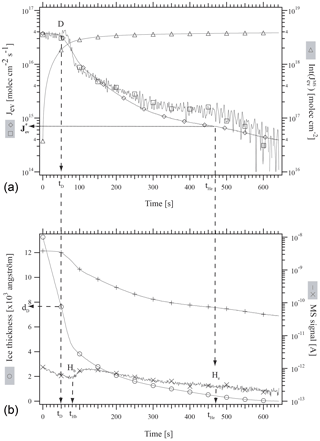Figure 1Typical experimental protocol of the evaporation at 192 K of an approximately 1.2 µm thick ice film doped with 5.4×1014 molecules of HCl. This illustration corresponds to experiment 11 of Table 2. (∘): ice thickness monitored by QCM (Å), (): “apparent” H2O evaporative flux, ${J}_{\mathrm{ev}}^{\mathrm{QCM}}$ , monitored using QCM (molec cm−2 s−1), (+): I18 MS signal for H2O, (×): I36 MS signal for HCl (A), (): J${}_{\mathrm{ev}}^{\mathrm{18}}$ evaporative flux calculated from I18 (molec cm−2 s−1), (Δ): Int(J${}_{\mathrm{ev}}^{\mathrm{18}}\right)$ time integral of J${}_{\mathrm{ev}}^{\mathrm{18}}$ (molec cm−2).

At t=0, the system is set from stirred flow to dynamic pumping that starts the evaporation experiment. The continuous curve marked with the empty squares symbol in Fig. 1a corresponds to ${J}_{\mathrm{ev}}^{\mathrm{QCM}}$, the evaporative flux of H2O calculated from the raw signal at the output of the QCMB. The diamond symbol () corresponds to ${J}_{\mathrm{ev}}^{\mathrm{18}}$ evaluated from I18, the MS signal amplitude for H2O monitored at $m/e=\mathrm{18}$. Int(${J}_{\mathrm{ev}}^{\mathrm{18}}\right)$ marked by triangles in Fig. 1a is the time integral of ${J}_{\mathrm{ev}}^{\mathrm{18}}$ and corresponds to the total number of H2O molecules that have evaporated from the ice film at t. D is the label at time tD at which Jev(H2O) decreased from its original value corresponding to pure ice to 85 % of its original value at t=0, and dD is the remaining thickness of the ice film at tD. Hb and He in Fig. 1b correspond to the time when HCl evaporation begins and ceases to be observed, respectively, using gas-phase residual mass spectrometry (× symbols in Fig. 1b), and are labeled tHb and tHe. The data have been treated in analogy to HNO3-doped ice through the formalism given in Delval and Rossi (2005). Akin to HNO3 the mass balance between HCl deposited, ${N}_{\mathrm{HCl}}^{\mathrm{dep}}$, and HCl recovered during ice evaporation, ${N}_{\mathrm{HCl}}^{\mathrm{evap}}$, agrees to within less than a factor of 2 under dynamic pumping conditions. We therefore estimate the average uncertainty (2σ) of the HCl mole fraction χHCl of ±18 % from the average discrepancy between ${N}_{\mathrm{HCl}}^{\mathrm{dep}}$ and ${N}_{\mathrm{HCl}}^{\mathrm{evap}}$ displayed in Table 2. In the following NHCl will always refer to ${N}_{\mathrm{HCl}}^{\mathrm{dep}}$ derived from the measurement of HCl at deposition because it refers to a directly measured quantity originating from a measured pressure decrease in a given volume and time interval ΔP∕Δt. The present experiments cover the evaporation of a small albeit important fraction of the model ice film for which the decrease in Jev(H2O) is significant.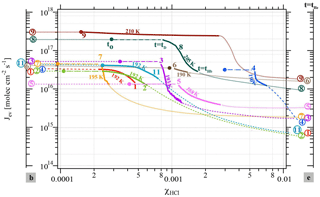Figure 2Change in the evaporative flux Jev(H2O) as a function of the HCl mole fraction (χHCl) for the cases presented in Table 2 color-coded according to the corresponding experiment number in Table 2. The colored and circled numbers on axis “b” (left) correspond to Jev(H2O) of pure ice before HCl deposition; the ones on axis “e” (right) are Jev(H2O) at t=tHe at the end of HCl evaporation. The colored circles in the data field mark the value of Jev(H2O) after HCl deposition at t=t0 and are equal to Jev(H2O) of pure ice. The start of any particular Jev(H2O) curve as a continuous solid (bold) line occurs at t=tD at 85 % of Jev(H2O) at t=0 (pure ice value, colored dot or circled number on axis “b” to the left) and ends at tHb, the beginning of HCl evaporation as displayed in Fig. 1b.

3 Results

The experimental data reported in Table 2 on the isothermal change in the evaporative flux of water, Jev(H2O), as a function of the average mole fraction of HCl, χHCl, in the remaining ice film during the evaporation process under dynamic conditions, are presented in Fig. 2. Dynamic pumping conditions ensure the absence of any readsorption of H2O vapor during evaporation owing to the low H2O partial pressures in the reactor. The axes labeled “b” and “e” correspond to the values of Jev(H2O) at the end of ice film deposition and after desorption of most of the adsorbed HCl from the HCl-doped ice film at tHe, respectively, as displayed in Fig. 1b. The average mole fraction χHCl of HCl in the remaining ice film as a function of time is calculated according to Delval and Rossi (2005). The change in χHCl owing to H2O evaporation is evaluated between t=tD and t=tHb, which corresponds to the time interval when the number of adsorbed HCl molecules is constant, as no release of HCl is observable in the gas phase at $m/e=\mathrm{36}$ before tHb. Table 2 also displays the initial value of the HCl mole fraction, ${\mathit{\chi }}_{\mathrm{HCl}}^{\mathrm{0}}$, calculated for the ice film just at the end of HCl deposition and marked by a colored circle on the experimental trajectory of a color-coded evaporating ice film displayed in Fig. 2. The average mole fraction of HCl in the ice film, χHCl, increases owing to evaporation of H2O from the ice film without loss of HCl such that the elapsed time increases with χHCl in Fig. 2.

The beginning of an evaporation experiment after the end of HCl doping (t=0 in Fig. 1 or t0 in Fig. 2) is marked by a colored circle of a given experiment whose parameters are displayed in Table 2 and Fig. 2 (see experiment 8). As pointed out above, at t=tD Jev(H2O) has decreased to an arbitrarily chosen value of 85 % of its original value measured at t=t0 that corresponds to the beginning of the bold color-coded smooth curve of a given experiment. Figure 2 essentially displays trajectories of evaporation experiments from t0 (colored circle) moving to tD and finishing at tHb between the two limiting values for pure ice (color coded number of a given experiment on axis “b” for “beginning”) and the remaining ice film at the end of measurable HCl desorption tHe (color-coded number of experiment on axis “e” for “Halogen end”). The trajectory of an experiment with values of χHCl between t0 (colored circle at ${\mathit{\chi }}_{\mathrm{HCl}}^{\mathrm{0}}\right)$ and tD (beginning of bold colored line, see experiment 8 in Fig. 2) ending at tHb (end of bold line, experiment 8) is presented as a bold dashed-dotted and bold smooth line from t0 to tHb, respectively, in order to emphasize the quantitative portion of the experiment. Thinner (color-coded) dotted lines connect the end of ice film deposition (colored circle on axis “b”) and HCl dosing with t0, the beginning of the evaporation experiment, and also describe the post phase of evaporation starting at tHb to tHe, in order to guide the eye of the reader to imagine a complete evaporation cycle.Figure 3Synopsis of the dependence of the evaporation range parameter rb∕e on the rate of deposition RHCl of HCl for temperatures between 188 and 210 K. Each point is marked with the total number of HCl molecules (NHCl) deposited on the ice film, the temperature of the ice film at HCl deposition and the experiment number (bold) referring to Table 2. The hashed area encompasses rb∕e values for dataset B (experiments 3, 4, 7, and 8). The color code goes from low (blue) over medium (green) to high (red) temperatures.

Two different data sets of the change in Jev(H2O) with χHCl may be distinguished in Fig. 2. The first kind of data set corresponds to the curves describing Jev for experiments 1, 2, 9 and 11 and is called dataset A. These traces present a slow continuous decrease in Jev(H2O) as χHCl increases during H2O evaporation. The second type of dataset shows an initial plateau of Jev(H2O) with increasing χHCl starting at the value of pure ice evaporation followed by a sudden decrease in Jev(H2O) and is found for experiments 3, 4, 7 and 8, which we call dataset B. Akin to HNO3, we have evaluated the impact of the HCl deposition protocol on the evaporation range parameter, rb∕e, which is the ratio between the evaporative flux of H2O at the beginning of ice evaporation, ${J}_{\mathrm{ev}}^{\mathrm{b}}$(H2O) reported on the left axis “b” in Fig. 2, and Jev(H2O) close to the end of the desorption of HCl, ${J}_{\mathrm{ev}}^{\text{e}}$(H2O), at t=tHe (the right axis “e” in Fig. 2). It describes the factor by which Jev(H2O) decreases within the limits of “b” and “e”. The impacts of both the rate of deposition of HCl on ice, RHCl, and its time integral corresponding to the dose of deposited HCl, NHCl, are presented in Figs. 3 and A1 (Appendix), respectively.

It appears from these figures that we have not succeeded in finding a simple experimental parameter that controls Jev(H2O), either with elapsed time or amount of adsorbed HCl expressed as the time dependence of χHCl. Instead, the data may roughly be classified along the two cases presented above, namely datasets A and B. The distinction between both data sets seems to be the rate of change (slope) in Jev(H2O) within a fairly narrow range of χHCl. Indeed, the available number of experiments clearly shows two distinct and limiting cases, whereas the search for other controlling parameters such as RHCl, NHCl and the temperature of deposition (Tice) for dataset A failed, akin to a similar HNO3 study (Delval and Rossi, 2005).

One may take note for instance of the low value of χHCl at 210 K for experiment 9 where the conditions of deposition are similar to experiments 1 and 2; however, its respective values of rb∕e differ significantly from experiment 9 (Fig. 3). In contrast, for dataset B the rb∕e values are similar for the whole set and range from 20 to 27.2, staying within a fairly narrow band. Moreover, they seem to be independent of RHCl and NHCl as for dataset A. In contrast, the rb∕e values for dataset A seem widely scattered over the explored parameter space. We have also investigated the impact of the deposition protocol on dD, which is the thickness of ice that is affected by the presence of HCl, namely the remaining thickness of ice whose Jev(H2O) value has decreased to 85 % of Jev(H2O) of pure ice. The results on dD as a function of RHCl and ${N}_{\mathrm{HCl}}^{\mathrm{dep}}$ are presented in Figs. 4 and A2 (Appendix), respectively. Taking the results of Figs. 3, 4, A1 and A2 together, we arrive at the following two conclusions.

1. Tice, RHCl and ${N}_{\mathrm{HCl}}^{\mathrm{dep}}$ are not controlling parameters or predictors for Jev(H2O) of either set.

2. The evaporation range parameters rb∕e and dD are not characterizing set A. In contrast, for dataset B, rb∕e and dD values fall into a narrow range with values varying from 460.7 to 636.0 nm compared to the original ice thickness d0 of 1500 nm or so (exact numbers in Table 2).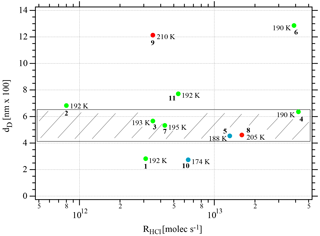Figure 4Synopsis of the dependence of dD on the rate of deposition RHCl of HCl for temperatures between 188 and 210 K. Each point is marked with the total number of HCl molecules (NHCl) deposited on the ice film, the temperature of the ice film at HCl deposition and the experiment number (bold font) referring to Table 2. The hashed area encompasses dD values for dataset B (experiments 3, 4, 7, and 8). The color code goes from low (blue) over medium (green) to high (red) temperatures.

4 Discussion

Figure 1 displays the evaporation history of sample 11 as an example whose deposition parameters are listed in Table 1. The initial average mole fraction ${\mathit{\chi }}_{\mathrm{HCl}}^{\mathrm{0}}$ of HCl, once deposition on the 1.44 µm thick ice film under stirred flow reactor conditions is terminated, has been estimated from the total number of H2O molecules contained in the ice film and the measured number of deposited HCl molecules, ${N}_{\mathrm{HCl}}^{\mathrm{dep}}$, for experiment 11 (Table 2). Table 2 and Fig. 1 reveal that for approximately 2.2×1018 H2O molecules in the film and 5.4×1014 molecules of deposited HCl, we obtain ${\mathit{\chi }}_{\mathrm{HCl}}^{\mathrm{0}}=\mathrm{2.7}×{\mathrm{10}}^{-\mathrm{4}}$. This HCl mole fraction represents an average value that takes into account all H2O molecules contained in the ice film, whereas in reality there will be a HCl gradient across the ice film, as has been observed in the case of the HNO3/ice system (Delval and Rossi, 2005).

After the HCl deposition process on the typically 1.5 µm thick ice film the gate valve is opened in order to initiate the isothermal evaporation experiment under dynamic pumping conditions. Initially, H2O evaporates at fluxes Jev(H2O) that are characteristic of pure ice measured previously (Delval and Rossi, 2004; Pratte et al., 2006). These initial values ${J}_{\mathrm{ev}}^{\text{b}}$(H2O) are displayed on the left-hand “b” (= beginning) axis in Fig. 2. As the evaporation proceeds Jev(H2O) slightly decreases with time, as displayed in Fig. 1a, to the arbitrarily chosen point where Jev(H2O) has decreased to 85 % of the initial pure ice value, at which point the remaining ice thickness dD has decreased by approximately one-third to 771.7 nm remaining ice thickness as displayed in Fig. 1b and Table 2. Further evaporation of H2O leads to a continuous decrease in Jev(H2O) at a corresponding increase in χHCl up to point Hb defined above (“Halogen beginning”) at tHb (Fig. 1b) where HCl starts to desorb from the ice film as monitored using the residual MS signal at $m/e=\mathrm{36}$.

For t<tHb, χHCl is given by the number of originally deposited HCl molecules that remain adsorbed on the ice film up to tHb and the remaining H2O molecules in the film. In contrast, for t>tHb the composition of the remaining ice film must be determined by taking into account the loss by evaporation of both H2O and HCl. The present experimental configuration is not adapted to quantitatively measure HCl loss. Therefore, we have chosen to display the temporal development of Jev(H2O) for t<tHb in Fig. 2 as a function of the average value of the HCl mole fraction χHCl. However, the value of Jev(H2O) at t=tHe where most of the HCl has desorbed from the ice film is plotted on the right axis labeled “e” (= end) as ${J}_{\mathrm{ev}}^{\text{e}}$(H2O) in Fig. 2 in order to provide a limit for the minimum value of the evaporation rate Jev(H2O) at an ice film thickness dHe of approximately 80±10 nm as displayed in Fig. 1b. We have observed in the past that Jev(H2O) for a pure ice film of an approximate thickness of 80 nm or less also slows down, presumably owing to island formation at the very end of pure thin ice film evaporation (Delval and Rossi, 2005). Therefore, results are becoming more difficult to interpret, such that we halted the experiment at tHe. The ratio ${r}^{\mathrm{b}/\mathrm{e}}={J}_{\mathrm{ev}}^{\text{b}}\left({\mathrm{H}}_{\mathrm{2}}\mathrm{O}\right)/{J}_{\mathrm{ev}}^{\text{e}}\left({\mathrm{H}}_{\mathrm{2}}\mathrm{O}\right)$ is displayed in Table 2 and is an operational evaporation range parameter that estimates the extent of decrease in Jev(H2O) for a thick HCl-doped ice film of µm size down to thicknesses of approximately 80 nm.

At the start of the evaporation experiment the equilibrium vapor pressure of H2O, Peq(H2O), is that of pure ice (Delval et al., 2003; Delval and Rossi, 2004; Pratte et al., 2006) owing to the small values of ${\mathit{\chi }}_{\mathrm{HCl}}^{\mathrm{0}}$. Raoult's law applies to such small values of χHCl but leads to unmeasurably small deviations from the observed vapor pressure of H2O which is that of pure ice. In fact, we have never observed an equilibrium vapor pressure that did not correspond to pure ice in the course of the present work that seems to be the consequence of the small average mole fractions of HCl in the H2O∕HCl system. This value of Peq(H2O) is observed throughout the evaporation up to tHe as the film is apparently sufficiently H2O-rich to support an equilibrium vapor pressure characteristic of pure ice consistent with the published, albeit revised, HCl∕H2O-phase diagram by Iannarelli and Rossi (2014). In view of the decreasing values of Jev(H2O) displayed in Fig. 2 the equilibrium vapor pressure of pure ice can only be maintained if the condensation rate coefficient kc for H2O adsorption decreases to the same extent as Jev(H2O), in agreement with previous work (Delval et al., 2003; Delval and Rossi, 2004; Pratte et al., 2006) and the concept of microscopic reversibility.

Figure 1a displays both the QCMB signal () as well as the corresponding MS signal for evaporating H2O at $m/e=\mathrm{18}$ (). Akin to the HNO3∕H2O system studied previously (Delval and Rossi, 2005) we obtain a perfect match between the two signals for t<tD, whereas for t>tD there is a significant discrepancy, especially at t>300 s, amounting to typically less than a factor of 2. Such a disagreement has been noted before for HNO3∕H2O, albeit to a larger extent. The reason for this behavior of the QCMB signal has not been studied in detail but may well lie in a structural rearrangement of the condensed phase during evaporation that will lead to a change in the calibration factor Cf defined in Table 1 and in Delval and Rossi (2005). In view of the straightforward interpretation of the calibrated MS signal at $m/e=\mathrm{18}$ we have used it for the measurement of Jev(H2O) at t>tD akin to the previous study on HNO3∕H2O.

The accuracy with which both tHb and tHe can be determined depends on the temporal change in the background MS signal for HCl at $m/e=\mathrm{36}$ displayed in Fig. 1b following the dosing of the thin ice film under stirred flow conditions. Figure 1b displays the MS signal at $m/e=\mathrm{36}$ as a function of time just before the start of HCl desorption at tHb that is signalled by an increase in the MS intensity, whereas tHe corresponds to the return of the HCl signal to the decaying HCl background in comparison to a reference experiment in which the HCl background was monitored as a function of time following the admission of the same HCl dose in the absence of an ice film. We estimate that tHb is determined to ±10 s, whereas tHe may only be estimated to ±100 s by virtue of the vanishing intensity of the HCl MS signal compared to its slowly decaying background.

Previous work has established that the rate of deposition of HCl, RHCl, in the range 1×1013 to 5×1013 molecule s−1 for the 0.78 cm2 surface area of the Si window leads to the formation of a crystalline HCl hydrate, HCl⋅xH2O, whereas values outside of this range seemed to favor the formation of an amorphous HCl∕H2O mixture (Delval et al., 2003). The exact nature of this undoubtedly crystalline solid is still unknown. However, IR spectroscopic work on hydroxonium salts of the type ${\mathrm{H}}_{\mathrm{3}}{\mathrm{O}}^{+}{\mathrm{X}}^{-}$ suggests that the ν1 and ν3 peak positions of the symmetric and antisymmetric O–H stretch vibrations must correspond to a molecular structure in which the distance between the cation and anion is unusually large (Desbat and Huong, 1975; Iannarelli and Rossi, 2016). Recent work has shown that the presence of HCl hexahydrate (HCl⋅6H2O) under the present experimental conditions could be safely excluded, however, the FTIR absorption spectrum clearly shows the presence of dissociated HCl within the ice film (Iannarelli and Rossi, 2014). Akin to HCl⋅6H2O that is known to nucleate with difficulty, crystallization of this unknown HCl hydrate seems to occur only under specific conditions of temperature and/or HCl deposition. Owing to the quantitative control of HCl deposition on the ice film in this work we infer the presence of at least two forms of HCl hydrates in the temperature range chosen in analogy to previous work (Delval et al., 2003).

We clearly point out that the present work has been performed without simultaneous spectroscopic control of the HCl/ice deposit that would have allowed the identification and/or quantification of the molecular composition of the condensate. Because we lack a spectroscopic probe for the ice film deposited on the QCMB in the present work, we are seeking a correlation between the type of HCl∕H2O deposit, either crystalline or amorphous, and the relevant HCl deposition parameters. Previous work has revealed a distinctly different temporal dependence of Jev(H2O) between the crystalline and amorphous HCl hydrates with the extent of H2O evaporation from the film, at both low (Delval et al., 2003) and high temporal resolution (Iannarelli and Rossi, 2014).

Datasets A and B have been characterized above in terms of a difference in the temporal dependence of Jev(H2O) as a function of increasing χHCl owing to H2O evaporation. Taking one example of each set, Fig. 2 reveals a distinct difference between experiments 7 (set B) and 11 (set A) performed at T=195 and 192 K, respectively, despite comparable HCl deposition parameters (Table 2). At t>tD Jev(H2O) for experiment 7 decreases at once with χHCl, in contrast to experiment 11, whose Jev(H2O) value gradually starts to decrease at roughly the same value of χHCl as experiment 7. In addition, in both cases the extent of the decrease in Jev(H2O) is roughly equal between tD and tHb within less than a factor of 2. Set B data are in marked contrast to set A independent of the magnitude of χHCl, which is highlighted by a comparison of experiments 11 (set A) and 4 (set B) at 192 and 190 K, respectively. The abrupt decrease in Jev(H2O) for set B as well as the gradual decline for set A, both at tD, occur before HCl starts to evaporate from the sample at tHb and appears therefore to be independent of χHCl within the range explored in the present work.

If we consider the mean value dD for dataset B (Figs. 4 and A2) we find 549.0±120.0 nm compared to the 1500 nm or so original ice thickness which corresponds to approximately 8.5×1017 molecules of H2O spread out over 0.50 cm2. These H2O molecules are impacted by the presence of HCl to some extent because Jev(H2O) is slowed down significantly compared to pure ice. Previous results (Delval et al., 2003) on the deposition of HCl on ice under conditions where the presence of an as yet unidentified crystalline hydrate HCl⋅xH2O was confirmed by FTIR absorption led to the conclusion that on average the amount of “trapped” H2O within dD corresponded to 1.2×1018 molecules starting with an original 1 µm thick ice film that was subsequently doped with HCl. This quantity of H2O, when scaled from the 0.78 cm2 area of the Si window used for FTIR absorption to the area of 0.5 cm2 of the QCMB, leads to 7.7×1017 H2O, which is in satisfactory agreement with the present measurement of dD or 8.5×1017 H2O in the present work. We may add that the previous value of 1.2×1018 H2O from the work of Delval et al. (2003) corresponding to dD obtained in that work has been derived using He–Ne interferometry, which is a crude method for measuring the film thickness.

Specifically, considering the low value of dD of experiments 1 and 10 (Table 2, Fig. 4), we may define the behavior of these condensates as “ice-like” because roughly 80 % of the ice sample of roughly 1.5 µm thickness has evaporated at Jev(H2O) of pure H2O ice before it slows down. This decrease in Jev(H2O) is a kinetic effect and acts on both the rate of evaporation as well as on the mass accommodation coefficient, the ratio of which remains constant because the characteristic vapor pressure of pure ice is maintained until t=tHe when the sample runs out of H2O and HCl. For sample 1 this conclusion is not too surprising owing to its extremely low HCl dose of 0.8 formal HCl monolayers. Sample 10 in comparison with the other members of dataset A allows us to conclude that dD is proportional to Tice for dataset A. Low temperatures prevent rapid diffusion of HCl into the bulk of the ice film, which leaves the majority of the total mass of the thin film deposited void of any HCl. Therefore, a large fraction of the total mass of the thin film deposit evaporates at values of Jev(H2O) characteristic of pure ice before it decreases to lower values when the presence of HCl slows down Jev(H2O). Although our experiment does not reveal the location of the thin layer of HCl-contaminated ice, plausibility suggests that it is located on top of the ice film at the gas-condensed interface. The corollary of this is that it is impossible to “cap” a pure ice sample with a thin layer of an atmospheric condensable gas of lower vapor pressure in the hope to lower the vapor pressure of the condensate or slow down H2O evaporation. This capping has been attempted many times, and examples abound. However, all attempts to lower the ice vapor pressure of the condensate using low amounts of polar contaminants of ice, such as HNO3, HCl or HBr, have proven futile to date (Biermann et al., 1998).

The other members of dataset A are examples (experiments 2, 9, and 11) with high values of dD at higher temperatures and higher HCl doses (Table 2). Because of higher presumed interfacial HCl concentrations these samples experience a decrease in Jev(H2O) owing to rapid diffusion of HCl into ice that affects the kinetics of evaporation to some depths of the ice film corresponding to higher values of dD. Both high HCl doses and high temperatures favor HCl contamination of deeper layers of the HCl film, and hence high values of dD.

Tentatively, we assign a crystalline, yet unknown molecular structure and stoichiometry to samples A in contrast to samples of dataset B that we identify with an amorphous structure in terms of a liquid HCl∕H2O mixture of variable composition. The main argument in favor of this assignment comes from recent kinetic work performed by Iannarelli and Rossi (2016a), who show that both Jev(H2O) as well as the corresponding mass accommodation coefficient or the adsorption rate coefficient for H2O adsorption are highly scattered for crystalline HCl hexahydrate, whereas the amorphous mixture shows a significantly smaller scatter of the experimental and thermodynamic values (Iannarelli and Rossi, 2014). Figures A3 and A4 in the Appendix show this substantial difference in experimental scatter for the amorphous HCl∕H2O mixture (Fig. A3) compared to crystalline HCl hexahydrate (Fig. A4).

Figure 3 displays the range parameter rb∕e as a function of RHCl for all data displayed in Table 2. It is noteworthy that rb∕e is in the range 20 to 27 for set B experiments 3, 4, 7 and 8 compared to set A data that seem to be scattered throughout the range. Members of dataset B show a common average range for both dD and rb∕e, which is the reason we tentatively assign these structures to amorphous liquid mixtures of high viscosity at the prevailing temperatures.

In conclusion, we take the simultaneous occurrence of the restricted range of the measured remaining thickness of ice ${d}_{D}=\mathrm{549.0}±\mathrm{120.0}$ nm together with a similarly restricted range of rb∕e between 20 and 27 as well as the substantial overlap in RHCl between the present and previous work (Delval et al., 2003) as an indication that set B evaporation experiments imply the presence of an amorphous HCl∕H2O mixture. In contrast, the scatter of the set A data across the range of rb∕e and dD values suggests the presence of an as yet unidentified crystalline HCl hydrate. If, and only if, the HCl deposition conditions rapidly establish thermodynamic equilibrium, experiment 2 (low HCl flow rate) lies in the “ice” region in the temperature interval 192–210 K, whereas experiment 11 (high HCl flow rate) should access crystalline HCl hexahydrate at 192 K but not at 210 K according to the revised HCl∕H2O-phase diagram of Iannarelli and Rossi (2014). It remains to be seen whether or not the published FTIR absorption spectrum in Delval et al. (2003) turns out to be identical to the expected crystalline HCl hexahydrate invoked as condensate in set A molecules, similar HCl deposition parameters notwithstanding. This proposal awaits further confirmation from FTIR spectroscopic work that will be combined in the future with the QCMB measurement. At this point we reiterate our earlier statement that Tice, RHCl, ${N}_{\mathrm{HCl}}^{\mathrm{dep}}$ do apparently not control Jev(H2O) of both datasets.

5 Atmospheric implications

The evaporation range parameter rb∕e may be used to quantitatively evaluate the upper limit of the evaporative lifetime extension of thin ice films under conditions of H2O vapor subsaturation. In the interest of applying the data of the present work to atmospheric conditions we make the assumption that typical atmospheric cirrus cloud particles of several µm diameter may be approximated by macroscopic thin films used to obtain the present data. The time tev in seconds to complete evaporation of an ice particle of radius r at a given relative humidity (rh) is given in Eq. (1) (Chiesa and Rossi, 2013; Iannarelli and Rossi, 2016a):

$\begin{array}{}\text{(1)}& {t}_{\mathrm{ev}}=\frac{{\left(\frac{\mathit{\rho }\phantom{\rule{0.125em}{0ex}}{N}_{L}}{M}\right)}^{\mathrm{2}/\mathrm{3}}\left(\frac{r}{a}\right)}{{J}_{\mathrm{ev}}\left(\mathrm{1}-\text{rh}\right)},\end{array}$

where ρ is the density of ice (0.916 and 0.925 g cm−3 at 273 and 173 K, respectively), M= 18 g mol−1 for H2O, r and a are the ice particle radius and the distance between two molecular layers in H2O(ice), respectively (Iannarelli and Rossi, 2016a). Equation (1) is based on a simple layer-by-layer evaporation model of H2O(ice) from a spherical ice particle following a zero-order rate law for Jev or a first order rate law for its inverse, namely H2O adsorption or condensation. For a 10 µm diameter ice particle approximated by thin film experiment 1 (Table 2) at rh = 80 %, T=192 K, ${J}_{\mathrm{ev}}=\mathrm{3}×{\mathrm{10}}^{\mathrm{16}}$ molecule s−1 cm−2 (Petrenko and Whitworth, 1999) and $a=\mathrm{4}×{\mathrm{10}}^{-\mathrm{8}}$ cm we obtain tev=2050 s or 34 min. This is the value for a pure ice particle as Jev(H2O) for pure ice has been used at the outset of the evaporation experiment and is a lower limit to the true evaporation time owing to the competition of mass transfer and heterogeneous chemistry (Seinfeld and Pandis, 1998). Using ${r}^{\mathrm{b}/\mathrm{e}}=\mathrm{43}$ for experiment 1 tev is calculated to be 15 min and 24 h for a 100 nm and 10 µm diameter particle, respectively, whereas the evaporative lifetime of an analogous pure ice particle would be only 21 s for the 100 nm diameter pure ice particle. Cirrus ice particles are frequently in the lower tens of µm size range resulting in a longer evaporation time considering that the simple evaporation model scales linearly with the radius of the ice particle. In conclusion we may state that, owing to the lifetime extension of ice particles contaminated by HCl, HNO3 or other volatile atmospheric trace gases such as HOCl, HOBr or HONO, small particles may have a chance to survive subsaturated regions of the atmosphere so as to function as cloud condensation or ice nuclei for the following cloud cycle (Delval and Rossi, 2004, 2005; Pratte et al., 2006).

We would like to stress that the variable rb∕e factor displayed in Table 2 leads to a significant increase in the evaporative lifetime of a contaminated ice particle and amounts to a kinetic effect that does not affect the equilibrium vapor pressure of the ice particle in question: it is that of pure ice from the start of the evaporation experiment to t=tHe and therefore affects both the rate of evaporation and accommodation equally. However, in cases where the sample has lost most of its mass, the vapor pressure decreases and becomes somewhat uncertain. In the present case the above statement is correct for t=tHb, that is, before halogen evaporation. Of note is the fact that the accommodation coefficient α is frequently less than unity, in contrast to what is often assumed, which will lower the rate of evaporation for pure ice, hence increasing the evaporative lifetime of pure ice particles for T≥180 K, as proposed in previous work (Delval and Rossi, 2004, 2005; Pratte et al., 2006).

As a token example of the potential atmospheric importance of the measured evaporative lifetimes of ice particles laced with condensable atmospheric trace gases, we may take the formation, persistence and evaporation of contrails and cirrus clouds in the UT/LS. These are ice clouds forming on non-volatile ice nuclei at the corresponding temperature and relative humidity conditions and that also frequently serve as reaction sites for heterogeneous atmospheric reactions in connection with ozone depletion and chlorine activation chemistry in the LS. Under certain conditions, Schumann and coworkers used the concept of the increase in the evaporative lifetimes of contaminated ice particles in aviation contrails occurring mostly in the UT, but sometimes also in the LS, in order to explain the persistence of ice clouds below ice saturation conditions up to a certain time duration. Ice clouds have a significant radiative forcing effect that is of interest in evaluating the climate forcing of high-flying aircraft in future aviation scenarios (Lewellen, 2014; Schumann et al., 2017a, b). However, the results of the present work show that the rate of evaporation of ice films doped with small amounts of acidic trace gases significantly slows down in a complex manner over the evaporation history of the film or particle, and that the application of Eq. (1) to atmospheric situations should be carried out with caution.

6 Conclusions

Despite the scatter of the values of rb∕e and dD in dataset A displayed in Figs. 3 and 4 and the apparent lack of influence of the deposition parameters (Tice, RHCl, on Jev(H2O), we may state several key points from the present work.

• a.

We observe two types of behavior, both complex, as far as the temporal change in Jev(H2O) with ongoing evaporation of H2O from a HCl∕H2O condensate is concerned. We have named it sets A and B that represent limiting behavior as not all performed experiments fit into this scheme.

• b.

At low temperature or low dose of deposited HCl $\left({N}_{\mathrm{HCl}}^{\mathrm{dep}}\right)$ set A samples, especially samples 1 and 10, reveal an “ice-like” behavior that corresponds to a low value of dD. This means that the HCl∕H2O condensate evaporates a large fraction of the sample thickness at a value of Jev(H2O) characteristic of pure ice before slowing down at an increasing mole fraction of HCl upon H2O evaporation. This corresponds to a two-phase system consisting of a major ice-like and minor HCl∕H2O phase, both with significantly different values of Jev(H2O).

• c.

High values of dD are observed at high Tice or ${N}_{\mathrm{HCl}}^{\mathrm{dep}}$ values for set A samples. This means that the sample evaporates H2O at Jev(H2O) characteristic of pure ice for a relatively short time of its evaporation history because the quantity of HCl is sufficient to decrease Jev(H2O) already at high values of dD by rapidly diffusing to deeper layers of the ice film. An equivalent way of expressing the point would be to state that dD which is an indicator of the total mass of the ice film, is proportional to Tice for Set A.

• d.

Set A samples generally show scattered values of both dD and rb∕e values that we attribute to the existence of a two-phase binary system, namely a pure ice phase and a crystalline HCl hydrate phase of as yet unknown stoichiometry HCl⋅xH2O, but probably HCl hexahydrate. At first the pure ice phase starts to evaporate as a whole for a fairly long time at characteristic values of Jev(H2O) until the pure ice phase has disappeared, followed by the crystalline HCl∕H2O phase at a lower rate of Jev(H2O) to attain the characteristic value for the evaporation of the crystalline HCl⋅xH2O phase.

• e.

Set B samples are tentatively identified as single-phase binary amorphous mixtures of HCl∕H2O whose kinetic properties are uniform and thus fairly independent of the HCl concentration at the gas-condensed phase interface. The observation of a medium-sized average value for both rb∕e and dD is consistent with these observations and manifests itself as a continuous yet gradual decrease in Jev(H2O) with increasing χHCl. It is in distinct contrast to Set A, where Jev(H2O) values are those of pure ice until the ice phase has completely evaporated followed by a gradual decline of Jev(H2O) when the crystalline HCl hydrate starts to decompose.

• f.

It must be recalled that the vapor pressure of H2O remained that of pure ice during most of the thickness of the H2O∕HCl condensate down to approximately 80 nm, at which point we halted the evaporation experiment. This result is expected based on Raoult's law owing to the small average HCl mole fractions in doped ice used in the present work: it would make the decrease in the H2O saturation vapor pressure unmeasurably small. The present results therefore primarily address the kinetics of H2O evaporation which changes with the total mass of the thin film condensate and the concomitant increase in HCl concentration and/or mole fraction.

Data availability

Additional details on raw data may be found in the PhD thesis of Delval (2005). The URL from which the publication may be retrieved is at: http://doc.rero.ch/record/4686 (Delval, 2004).

Appendix A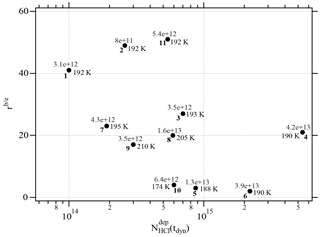Figure A1Graph of the dependence of the evaporation range parameter, rb∕e, on the number of adsorbed HCl, ${N}_{\mathrm{HCl}}^{\mathrm{dep}}$, adsorbed on ice for temperatures between 188 and 210 K. Each point is marked with the deposition rate of HCl molecules in molec s−1 on the ice film, the temperature of the ice film and the experiment running number (bold) referring to Table 2.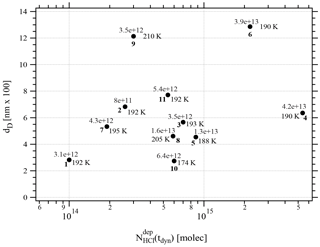Figure A2Graph of the dependence of the remaining thickness dD on the number of adsorbed HCl, ${N}_{\mathrm{HCl}}^{\mathrm{dep}}$, dispensed on ice for temperatures between 188 and 210 K. Each point is marked with the deposition rate of HCl in molec s−1 on the ice film, the temperature of the ice film and the experiment running number (bold) referring to Table 2.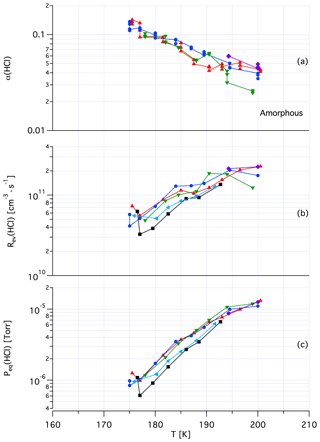Figure A3Synopsis of kinetic and thermodynamic results for an amorphous H2O∕HCl mixture using HCl as a probe gas. The symbols/colors used correspond to different experimental runs and the graphs show the scatter of the individual measurements within a series. Original data are published in Iannarelli and Rossi (2014).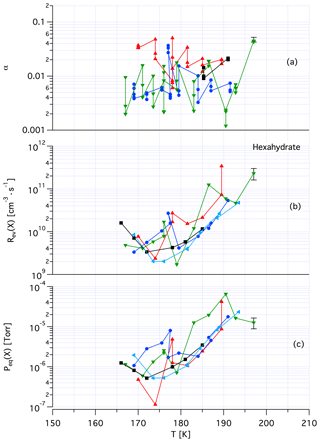Figure A4Synopsis of kinetic and thermodynamic results for crystalline HCl hexahydrate (HH) using X=HCl as a probe gas. The symbols/colors used correspond to different experimental runs and the graphs show the scatter of the individual measurements within a series. Original data are published in Iannarelli and Rossi (2014).

Table A1Brief summary of the amount of a molecular monolayer (coverage) of HCl adsorbed on H2O ice.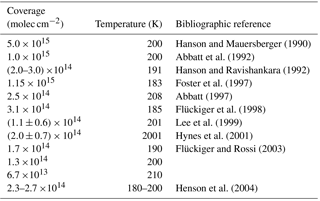Author contributions

CD performed all measurements and evaluated the data. MJR evaluated the data and wrote the paper.

Competing interests

The authors declare that they have no conflict of interest.

Acknowledgements

We sincerely thank Riccardo Iannarelli for Figs. A3 and A4 displayed in the Appendix. We also would like to thank the Swiss National Science Foundation (SNSF) for unfailing support over the years. This work has been performed under SNSF grant nos. 20-65299.01 and 200020-105471.

Edited by: Daniel Knopf
Reviewed by: J. Paul Devlin and one anonymous referee

References

Abbatt, J. P. D.: Interaction of HNO3 with water-ice surface at temperatures of the free troposphere, Geophys Res. Lett., 24, 1479–1482, 1997.

Abbatt, J. P. D.: Interactions of atmospheric trace gases with ice surfaces: Adsorption and reactions, Chem. Rev., 103, 4783–4800, 2003.

Abbatt, J. P. D., Beyer, K. D., Fucaloro, A. F., McMahon, J. R., Wooldridge, P. J., Zhang, R., and Molina, M. J.: Interaction of HCl vapor with water ice: implications for the stratosphere, J. Geophys. Res., 97, 15819–15826, 1992.

Banham, S. F., Horn, A. B., Koch, T. G., and Sodeau, J. R.: Ionisation and solvation of stratospherically relevant molecules on ice films, Faraday Discuss., 100, 321–332, 1995.

Biermann, U., Crowley, J. N., Huthwelker, T., Moortgat, G. K., Crutzen, P. J., and Peter, T.: FTIR studies on lifetime prolongation of stratospheric ice particles due to NAT coating, Geophys. Res. Lett., 25, 3939–3942, 1998.

Bolton, K. and Petterson, J. B. C.: Ice-Catalyzed Ionization of Hydrochloric Acid, J. Amer. Chem. Soc., 123, 7360–7363, 2001.

Bournel, F., Mangeney, C., Tronc, M., Laffon, C., and Parent, P.: Acidity of hydrogen chloride at the surface of low-temperature 40–150 K water-ice films, Phys. Rev. B, 65, 201404, https://doi.org/10.1103/PhysRevB.65.201404, 2002.

Buch, V., Dubrovskij, A., Mohamed, F., Parinello, M., Sadlej, J., Hammerich, A. D., and Devlin, J. P.: Protonated Water HCl Hydrates as Model Systems for Protonated Water, J. Phys. Chem. A, 112, 2144–2161, 2008.

Buch, V., Sadlej, J., Aytemiz-Uras, N., and Devlin, J. P.: Ice-Catalyzed Ionization of Hydrochloric Acid, J. Phys. Chem. A, 106, 9374–9389, 2002.

Chiesa, S. and Rossi, M. J.: The metastable HCl⋅6H2O phase – IR spectroscopy, phase transitions and kinetic/thermodynamic properties in the range 170–205 K, Atmos. Chem. Phys., 13, 11905–11923, https://doi.org/10.5194/acp-13-11905-2013, 2013.

Chu, L. T., Leu, M.-T., and Keyser, L. F.: Uptake of HCI in Water Ice and Nitric Acid Ice Films, J. Phys. Chem., 97, 7779–7785, 1993.

Delval, C.: Study of the kinetics of condensation and evaporation of water vapor over atmospherically relevant pure and doped ice films a multiple diagnostic approach, Rero, 252 pp., available at: http://doc.rero.ch/record/4686 (last access: 6 November 2018), 2004.

Delval, C.: Fig. 2.16 in “Study of the kinetics of condensation and evaporation of water vapor over atmospherically relevant pure and doped ice films: a multiple diagnostic approach”, Ph.D. thesis no. 3159, Ecole Polytechnique Fédérale de Lausanne (EPFL), Lausanne, Switzerland, 234 pp., 2005.

Delval, C. and Rossi, M. J.: The kinetics of condensation and evaporation of H2O from pure ice in the range 173 to 223 K: A quartz crystal microbalance study, Phys. Chem. Chem. Phys., 6, 4665–4676, 2004.

Delval, C., Fluckiger, B., and Rossi, M. J.: The rate of water vapor evaporation from ice substrates in the presence of HCl and HBr: implications for the lifetime of atmospheric ice particles, Atmos. Chem. Phys., 3, 1131–1145, https://doi.org/10.5194/acp-3-1131-2003, 2003.

Delval, C. and Rossi, M. J.: The influence of monolayer amounts of HNO3 on the evaporation rate of H2O over ice at $\mathrm{179}\le T/K\le \mathrm{208}$: A quartz crystal microbalance study, J. Phys. Chem. A, 109, 7151–7165, 2005.

Delzeit, L., Rowland, B., and Devlin, J. P.: Infrared Spectra of HCl Complexed/Ionized in Amorphous Hydrates and at Ice Surfaces in the 15–90 K Range, J. Phys. Chem., 97, 10312–10318, 1993a.

Delzeit, L., Rowland, B., and Devlin, J. P.: Ice Surface Reactions with Acids and Bases, J. Phys. Chem., 97, 10312–10318, 1993b.

Delzeit, L., Powell, K., Uras, N., and Devlin, J. P.: Ice Surface Reactions with Acids and Bases, J. Phys. Chem. B, 101, 2327–2332, 1997.

Desbat, B. and Huong, P. V.: Spectres i.r. et Raman des sels d'hydroxonium ${\mathrm{H}}_{\mathrm{3}}{\mathrm{O}}^{+}{\mathrm{Cl}}^{-}$, ${\mathrm{H}}_{\mathrm{3}}{\mathrm{O}}^{+}{\mathrm{Br}}^{-}$ et ${\mathrm{H}}_{\mathrm{3}}{\mathrm{O}}^{+}{\mathrm{SbCl}}_{\mathrm{6}}^{-}$, Spectrochim. Acta A, 31, 1109–1114, 1975.

Devlin, J. P., Uras, N., Sadlej, J., and Buch, V.: Discrete stages in the solvation and ionization of hydrogen chloride adsorbed on ice particles, Nature, 414, 269–271, 2002.

Devlin, J. P. and Kang, H.: Comment on “HCl adsorption on ice at low temperature: a combined X-ray absorption, photoemission and infrared study” by P. Parent, J. Lasne, G. Marcotte and C. Laffon, Phys. Chem. Chem. Phys. 13, 7142, 2011, Phys. Chem. Chem. Phys., 14, 1048–1049, 2012.

Donsig, H. A. and Vickerman, J. C.: Dynamic and static secondary ion mass spectrometry studies of the solvation of HCl by ice, J. Chem. Soc. Faraday Trans., 93, 2755–2761, 1997.

Ferriso, C. G. and Hornig, D. F.: Infrared Spectra of Oxonium Halides and the Structure of the Oxonium Ion, J. Chem. Phys., 23, 1464–1468, 1955.

Flückiger, B. and Rossi, M. J.: Common Precursor-mediated Reaction Mechanism for the Heterogeneous Interaction of D2O, HCl, HBr and HOBr on Ice at low Temperatures, J. Phys. Chem. A, 107, 4103–4115, 2003.

Flückiger, B., Thielmann, A., Gutzwiller, L., and Rossi, M. J.: Real-Time Kinetics and Thermochemistry of the Uptake of HCl, HBr and HI on Water Ice in the Temperature Range 190 to 210 K, Ber. Bunsenges. Phys. Chem., 102, 915–928, 1998.

Flückiger, B. and Delval, C.: Unpublished observations on the behavior of dangling hydrogen bonds (dH) in the presence of HCl at T<120 K. In essence, the dH absorption intensity at 3396 cm−1 did not decrease in the presence of small HCl partial pressures on the order of $\mathrm{3}×{\mathrm{10}}^{-\mathrm{6}}$ Torr at ambient temperature or 4 ppb, 2002.

Foster, K. L., Tolbert, M. A., and George, S. M.: Interaction of HCl with Ice: Investigation of the Predicted Trihydrate, Hexahydrate, and Monolayer Regimes, J. Phys. Chem. A, 101, 4979–4986, 1997.

Gertner, B. J. and Hynes, J. T.: Molecular Dynamics Simulation of Hydrochloric Acid Ionization at the Surface of Stratospheric Ice, Science, 271, 1563–1566, 1996.

Gilbert, A. S. and Sheppard, N.: Infra-red Spectra of the Hydrates of Hydrogen Chloride and Hydrogen Bromide Absorption Bands of the ${\mathrm{H}}_{\mathrm{5}}{\mathrm{O}}_{\mathrm{2}}^{+}$ Species, J. Chem. Soc. Faraday Trans., 69, 1628–1642, 1973.

Graedel, T. E. and Keene, W. C.: Tropospheric budget of reactive chlorine, Global Biogeochem. Cy., 9, 47–77, 1995.

Graham, J. D. and Roberts, J. T.: Interaction of HCl with crystalline and amorphous ice: implications for the mechanisms of ice-catalyzed reactions, Geophys. Res. Lett., 22, 251–254, 1995.

Graham, J. D. and Roberts, J. T.: Formation of HCl⋅6H2O from ice and HCl under ultrahigh vacuum, Chemom. Intell. Lab. Systems, 37, 139–148, 1997.

Hanson, D. R. and Mauersberger, K.: HCl∕H2O Solid Phase Vapor Pressures and HCl Solubility in Ice, J. Phys. Chem., 94, 4700–4705, 1990

Hanson, D. R. and Ravishankara, A. R.: Investigation of the Reactive and Nonreactive Processes Involving ClONO2 and HCl on Water and Nitric Acid Doped Ice, J. Phys. Chem., 96, 2682–2691, 1992.

Henson, B. F., Wilson, K. R., Robinson, J. M., Noble, C. A., Casson, J. L., and Worsnop, D. R.: Experimental isotherms of HCl and H2O ice under stratospheric conditions, Connections between bulk and interfacial thermodynamics, J. Chem. Phys., 121, 8486–8499, 2004.

Houghton, J. T., Ding, Y., Griggs, D. J., Noguer, M., van der Linden, P. J., Dai, X., Maskell, K., and Johnson, C. A. (Eds.): Climate Change 2001: The Scientific Basis, Cambridge Univ. Press, New York 2001.

Hynes, R. G., Mössinger, J., and Cox, R. A.: The interaction of HCl with water-ice at tropospheric temperatures, Geophys. Res. Lett., 28, 2827–2830, 2001.

Iannarelli, R. and Rossi, M. J.: H2O and HCl trace gas kinetics on crystalline HCl hydrates and amorphous HCl  H2O in the range 170 to 205 K: the HCl  H2O phase diagram revisited, Atmos. Chem. Phys., 14, 5183–5204, https://doi.org/10.5194/acp-14-5183-2014, 2014.

Iannarelli, R. and Rossi, M. J.: The mid-IR absorption cross sections of α- and β-NAT (HNO3⋅3H2O) in the range 170 to 185 K and of metastable NAD (HNO3⋅2H2O) in the range 172–182 K, J. Geophys. Res.-Atmos., 120, 11707–11727, 2016a.

Iannarelli, R. and Rossi, M. J.: Heterogeneous kinetics of H2O, HNO3 and HCl on HNO3 hydrates (α-NAT, β-NAT, NAD) in the range 175–200 K, Atmos. Chem. Phys., 16, 11937–11960, https://doi.org/10.5194/acp-16-11937-2016, 2016b.

Jensen, E. J., Toon, O. B., Vay, S. A., Ovarlez, J., May, R., Bui, T. P., Twohy, C. H., Gandrud, B. W., Pueschel, R. F., and Schumann, U.: Prevalence of ice-supersaturated regions in the upper troposphere: Implications for optically thin ice cloud formation, J. Geophys. Res., 106, 17253–17266, 2001.

Kang, H., Shin, T. H., Park, S. P., Kim, I. K., and Han, S. J.: Acidity of Hydrogen Chloride on Ice, J. Am. Chem. Soc., 122, 9842–9843, 2000.

Kong, X., Waldner, A., Orlando, F., Artiglia, L., Huthwelker, Th., Ammann, M., and Bartels-Rausch, Th.: Coexistence of Physisorbed and Solvated HCl At Warm Ice Surfaces, J. Phys. Chem. Lett., 8, 4757–4762, 2017.

Kuhs, W. F., Sippel, C., Falenty, F., and Hansen, C. T.: Extent and relevance of stacking disorder in “ice Ic”, P. Natl. Acad. Sci. USA, 109, 21259–21264, 2012.

Lee, S.-H., Leard, D. C., Zhang, R., Molina, L. T., and Molina, M. J.: The HCl+ClONO2 reaction on various water ice surfaces, Chem. Phys. Lett., 315, 7–11, 1999.

Leu, M.-T., Moore, S. B., and Keyser, L. F.: Heterogeneous Reactions of Chlorine Nitrate and Hydrogen Chloride on Type I Polar Stratospheric Clouds J. Phys. Chem., 95, 7763–7771, 1991.

Lewellen, D. C.: Persistent Contrails and Contrail Cirrus – Part II: Full Lifetime Behavior, J. Atmos. Sci., 71, 4420–4438, 2014.

Lu, Q. B. and Sanche, L.: Large enhancement in dissociative electron attachment to HCl adsorbed on ice via transfer of presolvated electrons, J. Chem. Phys., 115, 5711–5713, 2001.

Lundgren, J. O. and Olovson, I.: Hydrogen Bond Studies – XV. The Crystal Structure of Hydrogen Chloride Dihydrate, Acta Cryst., 23, 966–970, 1967.

Lundgren, J. O. and Olovson, I.: Hydrogen Bond Studies – XVI. The Crystal Structure of Hydrogen Chloride Trihydrate, Acta Cryst., 23, 971–976, 1967a.

Marcy, T. P., Fahey, D. W., Gao, R. S., Popp, P. J., Richard, E. C., Thompson, T. L., Rosenlof, K. H., Ray, E. A., Salawitch, R. J., Atherton, C. S., Bergmann, D. J., Ridley, B. A., Weinheimer, A. J., Loewenstein, M., Weinstock, E. M., and Mahoney, M. J.: Quantifying Stratospheric Ozone in the Upper Troposphere with in situ Measurements of HCl, Science, 304, 261–265, 2004.

Marti, J. and Mauersberger, K.: A survey and new measurements of ice vapor pressure at temperatures between 170 and 250 K, Geophys. Res. Lett., 20, 363–366, 1993.

Mauersberger, K. and Krankowsky, D.: Vapor pressure above ice at temperatures below 170 K, Geophys. Res. Lett., 30, 1121–1124, 2003.

Oppliger, R., Allanic, A., and Rossi, M. J.: Real-Time Kinetics of the Uptake of HOBr and BrONO2 on Ice and in the Presence of HCl in the Temperature Range 190–200 K, J. Phys. Chem. A, 101, 1903–1911, 1997.

Ortega, I. K., Escribano, R., Fernandez-Torre, D., Herrero, V. J., Maté, B., and Moreno, M. A.: The HCl hexahydrate: RAIR spectra and theoretical investigation, Chem. Phys. Lett., 396, 335–340, 2004.

Parent, P. and Laffon, C.: Adsorption of HCl on the Water Ice Surface Studied by X-ray Absorption Spectroscopy, J. Phys. Chem. B, 109, 1547–1553, 2005.

Parent, P., Lasne, J., Marcotte, G., and Laffon, C.: HCl adsorption on ice at low temperature: a combined X-ray absorption, photoemission and infrared study, Phys. Chem. Chem. Phys., 13, 7142–7148, 2011.

Parent, P., Lasne, J., Marcotte, G., and Laffon, C.: Reply to the “Comment on 'HCl adsorption on ice at low temperature: a combined X-ray absorption, photoemission and infrared study”' by J. P. Devlin and H. Kang, Phys. Chem. Chem. Phys. 2012, 14, https://doi.org/10.1039/c1cp22007a, Phys. Chem. Chem. Phys., 14, 1050–1053, 2012.

Petrenko, V. F. and Whitworth, R. W.: The Physics of Ice, Oxford University Press, 1999.

Pratte, P., van den Bergh, H., and Rossi, M. J.: The kinetics of H2O vapor condensation and evaporation on different types of ice in the range 130–210 K, J. Phys. Chem. A, 110, 3042–3058, 2006.

Schriver-Mazzuoli, L., Schriver A., and Hallou, A.: IR-reflection-absorption spectra of thin water ice films between 10 and 160 K at low pressure, J. Mol. Struct., 554, 289–300, 2000.

Schumann, U., Baumann, R., Baumgardner, D., Bedka, S. T., Duda, D. P., Freudenthaler, V., Gayet, J.-F., Heymsfield, A. J., Minnis, P., Quante, M., Raschke, E., Schlager, H., Vázquez-Navarro, M., Voigt, C., and Wang, Z.: Properties of individual contrails: a compilation of observations and some comparisons, Atmos. Chem. Phys., 17, 403–438, https://doi.org/10.5194/acp-17-403-2017, 2017a.

Schumann, U., Kiemle, C., Schlager, H., Weigel, R., Borrmann, S., D'Amato, F., Krämer, M., Matthey, R., Protat, A., Voigt, C., and Volk, C. M.: Long-lived contrails and convective cirrus above the tropical tropopause, Atmos. Chem. Phys., 17, 2311–2346, https://doi.org/10.5194/acp-17-2311-2017, 2017b.

Seinfeld, J. H. and Pandis, S. N.: Atmospheric Chemistry and Physics, from Air Pollution to Climate Change, John Wiley and Sons, Inc., 1998.

Solomon, S., Garcia, R. R., Rowland, F. S., and Wuebbles, D. J.: On the Depletion of Antarctic Ozone, Nature, 321, 755–758, 1986.

Solomon, S., Borrmann, S., Garcia, R. R., Portmann, R., Thomason, L., Poole, L. R., Winker, D., and McCormick, M. P.: Heterogeneous chlorine chemistry in the tropopause region, J. Geophys. Res.-Atmos., 102, 21411–21429, 1997.

Taesler, I. and Lundgren, J. O.: Hydrogen Bond Studies – CXXIX. An X-Ray Determination of the Crystal Structure of Hydrogen Chloride Hexahydrate, ${\mathrm{H}}_{\mathrm{9}}{\mathrm{O}}_{\mathrm{4}}^{+}{\mathrm{Cl}}^{-}\cdot \mathrm{2}{\mathrm{H}}_{\mathrm{2}}\mathrm{O}$, Acta Crystallogr. B, 34, 2424–2428, 1978.

Tolbert, M. A., Rossi, M. J., Malhotra, R., and Golden, D. M.: Reaction of Chlorine Nitrate with Hydrogen Chloride and Water at Antarctic Stratospheric Temperatures, Science, 238, 1258–1260, 1987.

Uras, N., Rahman, M., and Devlin, J. P.: Covalent HCl at the Surface of Crystalline Ice at 125 K: The Stable Phase at Submonolayer Levels, J. Phys. Chem. B, 102, 9375–9377, 1998.

WMO (World Meteorological Organization): Scientific Assessment of Ozone Depletion 2002, Global Ozone Research and Monitoring Project, report no. 47, Geneva, Switzerland, 2003.

Xueref, I. and Dominé, F.: FTIR spectroscopic studies of the simultaneous condensation of HCl and H2O at 190 K – Atmospheric applications, Atmos. Chem. Phys., 3, 1779–1789, https://doi.org/10.5194/acp-3-1779-2003, 2003.

Yoon, Y. K. and Carpenter, G. B.: The Crystal Structure of Hydrogen Chloride Monohydrate, Acta Cryst., 12, 17–20, 1959.

Zerefos, C. S., Eleftheratos, K., Balis, D. S., Zanis, P., Tselioudis, G., and Meleti, C.: Evidence of impact of aviation on cirrus cloud formation, Atmos. Chem. Phys., 3, 1633–1644, https://doi.org/10.5194/acp-3-1633-2003, 2003.